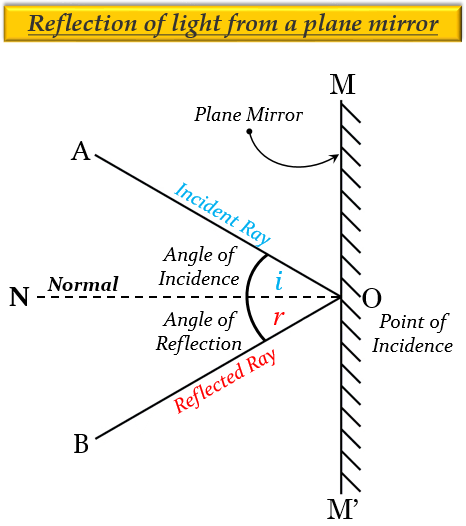# State and explain the laws of reflection of light at a plane surface (like a plane mirror), With the help of a labelled ray-diagram. Mark the angles of 'incidence' and 'reflection' clearly on the diagram. If the angle of reflection is 47.5°, what will be the angle of incidence?( a) The two laws of reflection are as follows:

1. The angle of incidence (∠i) is always equal to the angle of reflection (∠r), which is given as, ∠i = ∠rFor example, if we measure the angle of reflection NOB in the figure given above, we will find that it is exactly equal to the angle of incidence AON.

2. The incident ray, the reflected ray and the normal (at the point of incidence), all lie in the same plane. For example, in the figure given above, the incident ray AO, the reflected ray OB and the normal ON, all lie in the same plane.

(b) If the angle of reflection is 47.5°, the angle of incidence will also be 47.5°, because according to the first law of reflection, the angle of reflection is always equal to the angle of incidence (∠i = ∠r)

Updated on: 10-Oct-2022

110 Views